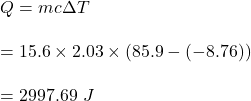## BRAINLIEST IF EXPLANATION IS GIVEN AND ANSWER IS CORRECT: How much energy is required to raise the temperature of 15.6 grams of

Question

BRAINLIEST IF EXPLANATION IS GIVEN AND ANSWER IS CORRECT:

How much energy is required to raise the temperature of 15.6 grams of ice from -8.76 degrees Celsius to 85.9 degrees Celsius? Use 3 sig figs.

in progress 0
2 weeks 2021-08-28T23:48:41+00:00 1 Answers 0 views 0

Q = 2997.69 J

Explanation:

Given that,

The mass of ice, m = 15.6 grams

The temperature increases from -8.76 degrees Celsius to 85.9 degrees Celsius.

The specifc heat of ice is 2.03 J/g°C

We need to find the amount of energy required. The formula for energy required is given by :So, the required heat is equal to 2997.69 J.